From: Richard, a student

I want to calculate 0.00353 to the power 1.3 How do I do it? I understand whole number powers.

Hi Richard.

 You've probably tried questions like this following one: What is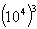?

Answer: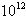, because you multiply the exponents.You've probably also tried questions that switch between exponent form and radical form:

 How can you re-write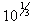? Answer: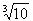Now put both these ideas together: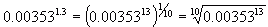Hope this helps,
Stephen La Rocque.